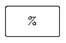## Percentages Assignment Help

Assignment Help: >> Introductory Mathematics - Percentages

Percentages:

Chapter percentage covers the conversion among percents, decimals & fractions.

CONVERT among percents, decimals, and fractions.

CALCULATE the percent differential.

A special application of proper fractions is the use of percentage.  While speaking of a 30% raise in pay, one is actually denoting a fractional part of a whole, 30/100.  A word percent means "hundredth;" therefore, 30% is based on the whole value being 100%. Therefore, to perform arithmetic operations, the 30% expression is represented as a decimal equivalent (0.30) rather than using the % form.

Calculator Usage, Special Keys

Percent KeyWhile pressed, the percent key divides the displayed number through 100.

 Changing a Percent to a Decimal Changing Common Fractions and Whole Numbers to Percent Changing Decimals to Percent Percent Differential Ratio